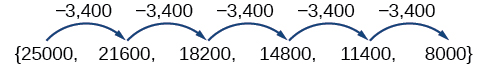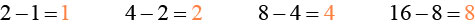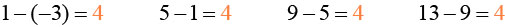# 13.2 Arithmetic sequences

 Page 1 / 8
In this section, you will:
• Find the common difference for an arithmetic sequence.
• Write terms of an arithmetic sequence.
• Use a recursive formula for an arithmetic sequence.
• Use an explicit formula for an arithmetic sequence.

Companies often make large purchases, such as computers and vehicles, for business use. The book-value of these supplies decreases each year for tax purposes. This decrease in value is called depreciation. One method of calculating depreciation is straight-line depreciation, in which the value of the asset decreases by the same amount each year.

As an example, consider a woman who starts a small contracting business. She purchases a new truck for $25,000. After five years, she estimates that she will be able to sell the truck for$8,000. The loss in value of the truck will therefore be $17,000, which is$3,400 per year for five years. The truck will be worth $21,600 after the first year;$18,200 after two years; $14,800 after three years;$11,400 after four years; and \$8,000 at the end of five years. In this section, we will consider specific kinds of sequences that will allow us to calculate depreciation, such as the truck’s value.

## Finding common differences

The values of the truck in the example are said to form an arithmetic sequence because they change by a constant amount each year. Each term increases or decreases by the same constant value called the common difference of the sequence. For this sequence, the common difference is –3,400.The sequence below is another example of an arithmetic sequence. In this case, the constant difference is 3. You can choose any term    of the sequence    , and add 3 to find the subsequent term.## Arithmetic sequence

An arithmetic sequence    is a sequence that has the property that the difference between any two consecutive terms is a constant. This constant is called the common difference    . If ${a}_{1}$ is the first term of an arithmetic sequence and $d$ is the common difference, the sequence will be:

$\left\{{a}_{n}\right\}=\left\{{a}_{1},{a}_{1}+d,{a}_{1}+2d,{a}_{1}+3d,...\right\}$

## Finding common differences

Is each sequence arithmetic? If so, find the common difference.

1. $\left\{1,2,4,8,16,...\right\}$
2. $\left\{-3,1,5,9,13,...\right\}$

Subtract each term from the subsequent term to determine whether a common difference exists.

1. The sequence is not arithmetic because there is no common difference.2. The sequence is arithmetic because there is a common difference. The common difference is 4.If we are told that a sequence is arithmetic, do we have to subtract every term from the following term to find the common difference?

No. If we know that the sequence is arithmetic, we can choose any one term in the sequence, and subtract it from the subsequent term to find the common difference.

Is the given sequence arithmetic? If so, find the common difference.

The sequence is arithmetic. The common difference is $–2.$

Is the given sequence arithmetic? If so, find the common difference.

The sequence is not arithmetic because $3-1\ne 6-3.$

## Writing terms of arithmetic sequences

Now that we can recognize an arithmetic sequence, we will find the terms if we are given the first term and the common difference. The terms can be found by beginning with the first term and adding the common difference repeatedly. In addition, any term can also be found by plugging in the values of $n$ and $d$ into formula below.

what are you up to?
nothing up todat yet
Miranda
hi
jai
hello
jai
Miranda Drice
jai
aap konsi country se ho
jai
which language is that
Miranda
I am living in india
jai
good
Miranda
what is the formula for calculating algebraic
I think the formula for calculating algebraic is the statement of the equality of two expression stimulate by a set of addition, multiplication, soustraction, division, raising to a power and extraction of Root. U believe by having those in the equation you will be in measure to calculate it
Miranda
state and prove Cayley hamilton therom
hello
Propessor
hi
Miranda
the Cayley hamilton Theorem state if A is a square matrix and if f(x) is its characterics polynomial then f(x)=0 in another ways evey square matrix is a root of its chatacteristics polynomial.
Miranda
hi
jai
hi Miranda
jai
thanks
Propessor
welcome
jai
What is algebra
algebra is a branch of the mathematics to calculate expressions follow.
Miranda
Miranda Drice would you mind teaching me mathematics? I think you are really good at math. I'm not good at it. In fact I hate it. 😅😅😅
Jeffrey
lolll who told you I'm good at it
Miranda
something seems to wispher me to my ear that u are good at it. lol
Jeffrey
lolllll if you say so
Miranda
but seriously, Im really bad at math. And I hate it. But you see, I downloaded this app two months ago hoping to master it.
Jeffrey
which grade are you in though
Miranda
oh woww I understand
Miranda
Jeffrey
Jeffrey
Miranda
how come you finished in college and you don't like math though
Miranda
gotta practice, holmie
Steve
if you never use it you won't be able to appreciate it
Steve
I don't know why. But Im trying to like it.
Jeffrey
yes steve. you're right
Jeffrey
so you better
Miranda
what is the solution of the given equation?
which equation
Miranda
I dont know. lol
Jeffrey
Miranda
Jeffrey
answer and questions in exercise 11.2 sums
how do u calculate inequality of irrational number?
Alaba
give me an example
Chris
and I will walk you through it
Chris
cos (-z)= cos z .
cos(- z)=cos z
Mustafa
what is a algebra
(x+x)3=?
6x
Obed
what is the identity of 1-cos²5x equal to?
__john __05
Kishu
Hi
Abdel
hi
Ye
hi
Nokwanda
C'est comment
Abdel
Hi
Amanda
hello
SORIE
Hiiii
Chinni
hello
Ranjay
hi
ANSHU
hiiii
Chinni
h r u friends
Chinni
yes
Hassan
so is their any Genius in mathematics here let chat guys and get to know each other's
SORIE
I speak French
Abdel
okay no problem since we gather here and get to know each other
SORIE
hi im stupid at math and just wanna join here
Yaona
lol nahhh none of us here are stupid it's just that we have Fast, Medium, and slow learner bro but we all going to work things out together
SORIE
it's 12
what is the function of sine with respect of cosine , graphically
tangent bruh
Steve
cosx.cos2x.cos4x.cos8x
sinx sin2x is linearly dependent
what is a reciprocal
The reciprocal of a number is 1 divided by a number. eg the reciprocal of 10 is 1/10 which is 0.1
Shemmy
Reciprocal is a pair of numbers that, when multiplied together, equal to 1. Example; the reciprocal of 3 is ⅓, because 3 multiplied by ⅓ is equal to 1
Jeza
each term in a sequence below is five times the previous term what is the eighth term in the sequence
I don't understand how radicals works pls
How look for the general solution of a trig functionByByBy OpenStaxBy OpenStaxBy Stephen VoronBy OpenStaxBy Samuel MaddenBy OpenStaxBy Anh DaoBy OpenStaxBy OpenStaxBy OpenStax